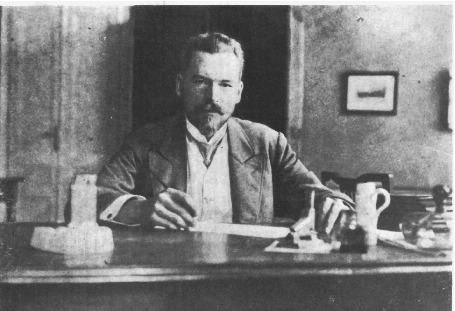#33rd M. Smoluchowski Symposium on Statistical Physics

3-4 December 2020
Europe/Warsaw timezone

## Diffusion-controlled reactions: Extension of time-dependent Smoluchowski’s rate coefficient to reactions in media with relaxation

3 Dec 2020, 18:17
1m
poster

### Speaker

Prof. Sergey Traytak (N.N. Semenov Federal Research Center for Chemical Physics, Russian Academy of Sciences)

### Description

Processes involving Brownian motion of small reactants toward much larger static particles (sinks) and subsequent trapping of these reactants by the sinks are very commonly encountered in both nature and in artificial media. Theoretically these processes are described by so-called trapping model of the irreversible bulk diffusion-controlled reactions. To avoid difficulties of classical diffusion theory the hyperbolic diffusion approximation is often used [1, 2]. Within the scope of the telegrapher equation the density distribution of reactants $\rho (r, t)$ about a spherical sink of radius $R$ obeys the initial boundary-value problem with Smoluchowski’s condition on the reaction surface . The primary task for the theory of diffusion-controlled reactions is the calculation of the reaction rate coefficient:
\begin{equation}
k\left(t\right) = k_S \frac{R}{\tau_d}\int_0^t \exp \left(-\frac{t-\zeta}{\tau_d}\right) \left. \frac{\partial \rho \left(r,\zeta\right)}{\partial r} \right| _{r\rightarrow R+} d\zeta .
\end{equation}
Hereafter $k_S=4\pi RD$ is the Smoluchowski steady state rate coefficient; $\tau_d$ and $D$ are the relaxation time for the gradients of density distribution of reactants and diffusion coefficient, respectively. We solved the posed problem exactly and, using expression (\ref{Tray1}), for the time-dependent reaction rate coefficient obtained
\begin{equation}
k \left(t\right) = k_S \left\lbrace \left[1-{\exp \left(- \frac{t}{\tau_d} \right)}\right] + \frac{R}{\sqrt{\tau_d D}}\left[ \exp \left( - \frac{t}{2\tau_d} \right) I_0\left(\frac{t}{2\tau_d} \right)-{\exp \left(- \frac{t}{\tau_d} \right)} \right] \right\rbrace ,
\end{equation}
where $I_0(z)$ is the first kind modified Bessel function of order zero. It is important to note that the last term in expression (1) was erroneously omitted in the relevant formula derived by Rice (see expression (289) in page 330 of book ). Formula (2) generalizes the classical Smoluchowski result to short time values, which is important for reactions occuring in media with relaxation .

 S. A. Rice, Diffusion-limited reactions, Amsterdam: Elsevier, 1985.
 D. Jou, J. Casas-Vazquez and G. Lebon, Extended irreversible thermodynamics (Fourth Edition), Springer, 2010.

### Primary author

Prof. Sergey Traytak (N.N. Semenov Federal Research Center for Chemical Physics, Russian Academy of Sciences)

### Presentation Materials

There are no materials yet.
###### Your browser is out of date!

Update your browser to view this website correctly. Update my browser now

×